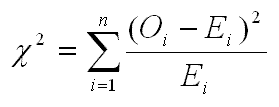The test statistic χ2 is calculated as below:n is the the number of observed and estimated values

Oi is the ith observed value

Ei is the ith estimated value